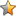اسم المؤلف
LOUIS KOMZSIK
التاريخ
4 سبتمبر 2017
المشاهدات
التقييمLoading...

سلسلة مايجب على كل مهندس معرفته عن العناصر المحددة
What Every Engineer Should Know about Computational Techniques of Finite Element Analysis 2nd ed
LOUIS KOMZSIK
Contents
Preface to the second edition xiii
Preface to the first edition xv
Acknowledgments xvii
I Numerical Model Generation 1
1 Finite Element Analysis 3
1.1 Solution of boundary value problems 3
1.2 Finite element shape functions . 6
1.3 Finite element basis functions 9
1.4 Assembly of finite element matrices . 12
1.5 Element matrix generation 15
1.6 Local to global coordinate transformation . 19
1.7 A linear quadrilateral finite element 20
References 29
2 Finite Element Model Generation 31
2.1 Bezier spline approximation . 31
2.2 Bezier surfaces 37
2.3 B-spline technology 40
2.4 Computational example . 43
2.5 NURBS objects 48
2.6 Geometric model discretization . 50
2.7 Delaunay mesh generation 51
2.8 Model generation case study . 54
References 57
3 Modeling of Physical Phenomena 59
3.1 Lagrange’s equations of motion . 59
3.2 Continuum mechanical systems . 61
3.3 Finite element analysis of elastic continuum 63
3.4 A tetrahedral finite element . 65
3.5 Equation of motion of mechanical system . 69
3.6 Transformation to frequency domain 71
viiviii
References 74
4 Constraints and Boundary Conditions 75
4.1 The concept of multi-point constraints . 76
4.2 The elimination of multi-point constraints . 79
4.3 An axial bar element . 82
4.4 The concept of single-point constraints . 85
4.5 The elimination of single-point constraints . 86
4.6 Rigid body motion support . 88
4.7 Constraint augmentation approach . 90
References 92
5 Singularity Detection of Finite Element Models 93
5.1 Local singularities 93
5.2 Global singularities 97
5.3 Massless degrees of freedom . 99
5.4 Massless mechanisms . 100
5.5 Industrial case studies 102
References 104
6 Coupling Physical Phenomena 105
6.1 Fluid-structure interaction 105
6.2 A hexahedral finite element . 106
6.3 Fluid finite elements . 109
6.4 Coupling structure with compressible fluid . 111
6.5 Coupling structure with incompressible fluid 112
6.6 Structural acoustic case study 113
References 115
II Computational Reduction Techniques 117
7 Matrix Factorization and Linear Systems 119
7.1 Finite element matrix reordering 119
7.2 Sparse matrix factorization . 122
7.3 Multi-frontal factorization 124
7.4 Linear system solution 126
7.5 Distributed factorization and solution . 127
7.6 Factorization and solution case studies . 130
7.7 Iterative solution of linear systems . 134
7.8 Preconditioned iterative solution technique 137
References 139ix
8 Static Condensation 141
8.1 Single-level, single-component condensation 141
8.2 Computational example . 144
8.3 Single-level, multiple-component condensation . 147
8.4 Multiple-level static condensation 152
8.5 Static condensation case study . 155
References 158
9 Real Spectral Computations 159
9.1 Spectral transformation . 159
9.2 Lanczos reduction 161
9.3 Generalized eigenvalue problem . 164
9.4 Eigensolution computation 166
9.5 Distributed eigenvalue computation . 168
9.6 Dense eigenvalue analysis 172
9.7 Householder reduction technique 175
9.8 Normal modes analysis case studies . 177
References 181
10 Complex Spectral Computations 183
10.1 Complex spectral transformation 183
10.2 Biorthogonal Lanczos reduction . 184
10.3 Implicit operator multiplication . 186
10.4 Recovery of physical solution 188
10.5 Solution evaluation 190
10.6 Reduction to Hessenberg form 191
10.7 Rotating component application . 192
10.8 Complex modal analysis case studies 196
References 199
11 Dynamic Reduction 201
11.1 Single-level, single-component dynamic reduction . 201
11.2 Accuracy of dynamic reduction . 203
11.3 Computational example . 206
11.4 Single-level, multiple-component dynamic reduction 208
11.5 Multiple-level dynamic reduction 210
11.6 Multi-body analysis application . 212
References 215
12 Component Mode Synthesis 217
12.1 Single-level, single-component modal synthesis . 217
12.2 Mixed boundary component mode reduction 219
12.3 Computational example . 222
12.4 Single-level, multiple-component modal synthesis . 225
12.5 Multiple-level modal synthesis 228x
12.6 Component mode synthesis case study . 230
References 232
III Engineering Solution Computations 235
13 Modal Solution Technique 237
13.1 Modal solution 237
13.2 Truncation error in modal solution . 239
13.3 The method of residual flexibility 241
13.4 The method of mode acceleration 245
13.5 Coupled modal solution application . 246
13.6 Modal contributions and energies 247
References 250
14 Transient Response Analysis 251
14.1 The central difference method 251
14.2 The Newmark method 252
14.3 Starting conditions and time step changes . 254
14.4 Stability of time integration techniques . 255
14.5 Transient response case study 258
14.6 State-space formulation . 259
References 262
15 Frequency Domain Analysis 263
15.1 Direct and modal frequency response analysis . 263
15.2 Reduced-order frequency response analysis . 264
15.3 Accuracy of reduced-order solution . 267
15.4 Frequency response case study . 268
15.5 Enforced motion application . 269
References 271
16 Nonlinear Analysis 273
16.1 Introduction to nonlinear analysis 273
16.2 Geometric nonlinearity 275
16.3 Newton-Raphson methods 278
16.4 Quasi-Newton iteration techniques . 282
16.5 Convergence criteria . 284
16.6 Computational example . 285
16.7 Nonlinear dynamics 287
References 288
17 Sensitivity and Optimization 289
17.1 Design sensitivity . 289
17.2 Design optimization . 290
17.3 Planar bending of the bar 294Contents xi
17.4 Computational example . 297
17.5 Eigenfunction sensitivities 302
17.6 Variational analysis 304
References 308
18 Engineering Result Computations 309
18.1 Displacement recovery 309
18.2 Stress calculation . 311
18.3 Nodal data interpolation . 312
18.4 Level curve computation . 314
18.5 Engineering analysis case study . 316
References 319
Annotation 321
List of Figures 323
List of Tables 325
Index 327
Closing Remark
كلمة سر فك الضغط : books-world.net